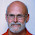## måndag 19 december 2016

### New Quantum Mechanics 21: Micro as Macro

The new quantum mechanics as realQM explored in this sequence of posts offers a model for the microscopic physics of atoms which is of the same form as the classical continuum mechanical models of macroscopic physics such as Maxwell's equations for electro-magnetics, Navier's equations for solid mechanics and Navier-Stokes equations for fluid mechanics in terms of deterministic field variables depending on a common 3d space coordinate and time.

realQM thus describes an atom with $N$ electrons realQM as a nonlinear system of partial differential equations in $N$ electronic wave functions depending on a common 3d space coordinate and time.

On the other hand, the standard model of quantum mechanics, referred to as stdQM, is Schrödinger's equation as a linear partial differential equation for a probabilistic wave function in $3N$ spatial coordinates and time for an atom with $N$ electrons.

With realQM the mathematical models for macroscopic and microscopic physics thus have the same form and the understanding of physics can then take the same form. Microphysics can then be understood to the same extent as macrophysics.

On the other hand, the understanding of microphysics according to stdQM is viewed to be fundamentally different from that of macroscopic physics, which effectively means that stdQM is not understood at all, as acknowledged by all prominent physicists.

As an example of the confusion on difference, consider what is commonly viewed to be a basic property of stdQM, namely that there is limit to the accuracy that both position and velocity can be determined on atomic scales, as expressed in Heisenberg's Uncertainty Principle (HUP).

This feature of stdQM is compared with the situation in macroscopic physics, where the claim is that both position and velocity can be determined to arbitrary precision, thus making the case that microphysics and microphysics are fundamentally different.

But the position of a macroscopic body cannot be precisely determined by one point coordinate, since  a macroscopic body is extended in space and thus occupies many points in space.  No one single point determines the position of and extended body. There is thus also a Macroscopic Uncertainty Principle (MUP).

The argument is then that if the macroscopic body is a pointlike particle,  then both its position and velocity can have precise values and thus there is no MUP. But a pointlike body is not a macroscopic body and so the argument lacks logic.

The idea supported by stdQM that the microscopic world is so fundamentally different from the macroscopic world that it can never be understood, thus may well lack logic. If so that could open to understanding of microscopic physics for human beings with experience from macroscopic physics.

If you think that there is little need of making sense of stdQM, recall Feynman's testimony:
• We have always had a great deal of difficulty understanding the world view that quantum mechanics represents. At least I do, because I’m an old enough man that I haven’t got to the point that this stuff is obvious to me. Okay, I still get nervous with it ... You know how it always is: every new idea, it takes a generation or two until it becomes obvious that there’s no real problem. I cannot define the real problem, therefore I suspect that there is no real problem, but I’m not sure there’s no real problem. (Int. J. Theoret. Phys. 21, 471 (1982).)
It is total confusion, if it is totally unclear if there is a problem or no problem and it is totally clear that nobody understands stdQM....

Recall that stdQM is based on a linear multi-dimensional Schrödinger equation, which is simply picked from the sky using black magic ad hoc formalism, which could be anything, and is then taken as a revelation about real physics when interpreted by reversing the black magics.

This is like scribbling down a sign/equation at random without intentional meaning, and then giving the sign/equation an interpretation as if it had an original meaning, which may well be meaningless, instead of expressing a meaning in a sign/equation to discover consequences and deeper meaning.

#### 1 kommentar:

1.Congratulations. I think you have made a great breakthrough in the understanding of QM, as with new theory of fligth (even I think I understand it).
How can this discovery be widespread?
Silence from the "Authorities" on QM is cause for concern. If your realQM is easy to debunk, it should have been critisized already.
Is it so that "silence is agreement"?